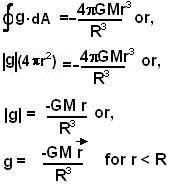## Sunday, September 14, 2008

### Irodov Problem 1.214

As in case of solutions to problems 1.212 and 1.211 we could use either the Gauss law of gravity or first consider the potential due to an infinitesimally small piece of the sphere and integrate it over the entire sphere. In this solution I shall use the Gauss law of gravity since it is much easier than the other way.

In the solution to problem 1.211 I have already computed the gravitational flux outside a uniform sphere and I shall not repeat it here. Please consult 1.211 for this part of the problem. In this solution I shall only consider the flux and potential inside the sphere.Considering the spherical symmetry in the problem it is clear that the gravitational flux vector g will always be directed radially and have the same magnitude at all points on a spherical surface that is concentric with the sphere. Thus, naturally our Gaussian surface will be a sphere concentric with the original sphere and passing through the point of interest (where the gravitational flux needs to be determined).
The area vector dA at any point on this Gaussian surface will also be directed radially (collinear with g).
The total mass contained within a Gaussian sphere of radius r will beThus from Gauss's law for gravity we have,Now the potential at a point r is given by,